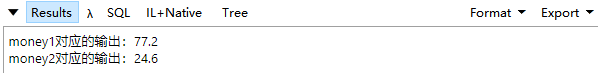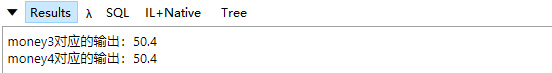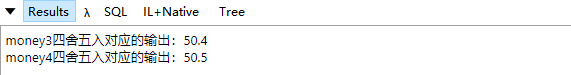2022
01-11

c语言中如何四舍五入?Math.Round的使用

decimal levyMoney1 = 77.24m;
decimal levyMoney2 = 24.56m;

//设置保留一位小数并输出
("money1对应的输出：" + Math.Round(levyMoney1, 1)).Dump();
("money2对应的输出：" + Math.Round(levyMoney2, 1)).Dump();decimal levyMoney3 = 50.35m;
decimal levyMoney4 = 50.45m;

//C#的Round函数都是采用Banker’s rounding（银行家算法）,即:四舍六入五取偶
("money3对应的输出：" + Math.Round(levyMoney3, 1)).Dump();
("money4对应的输出：" + Math.Round(levyMoney4, 1)).Dump();正确表达四舍五入

decimal levyMoney3 = 50.35m; decimal levyMoney4 = 50.45m; ("money3四舍五入对应的输出：" + Math.Round(levyMoney3, 1, MidpointRounding.AwayFromZero)).Dump(); ("money4四舍五入对应的输出：" + Math.Round(levyMoney4, 1, MidpointRounding.AwayFromZero)).Dump();